# Material Parameters Notes - Civil Engineering (CE)

## Civil Engineering (CE): Material Parameters Notes - Civil Engineering (CE)

The document Material Parameters Notes - Civil Engineering (CE) is a part of Civil Engineering (CE) category.
All you need of Civil Engineering (CE) at this link: Civil Engineering (CE)

Material parameters
In this section, we define the various material parameters and relate them to the Lam`e constants. We do this by defining the stress that is applied on the body in the shape of a cuboid. Since, the body is assumed to obey Hooke’s law, the state of strain gets fixed once the state of stress is specified because of the relation (6.74). Consequently, these parameters can be defined by prescribing the state of strain in the body as well.

Young’s modulus and Poisson’s ratio
Consider a cuboid being subjected to a uniform normal traction on two of its faces as shown in figure 6.3. Assuming the stress field is uniform, that is spatially constant, the Cartesian components of the stress at any point in the body is,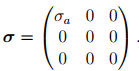(6.76)

Substituting the above in equation (6.74) for the stress we obtain the strain as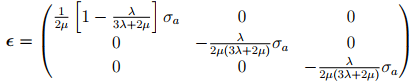(6.77)

Now, Young’s modulus, E, is defined as the ratio of the uniaxial stress to the component of the linearized strain along the direction of the applied uniaxial stress, i.e.,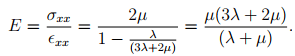(6.78)

The Poisson’s ratio , ν is defined as the negative of the ratio of the component of the strain along a direction perpendicular to the axis of loading, called the transverse strain to the component of the strain along the axis of loading, called the axial strain, i.e.,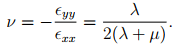(6.79)

In equations (6.78) and (6.79) we expressed the Young’s Modulus and Poisson’s ratio in terms of the Lam`e constants. This relation can be inverted to express Lam`e constants in terms of E and ν as,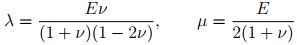(6.80)

Finally, substituting equation (6.80) in equation (6.73) and (6.74), the constitutive relations can be written in terms of the Young’s modulus and Poisson’s ratio as,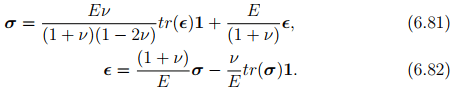Shear Modulus
Consider a cuboid being subjected to uniform pure shear stress of the form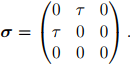(6.83)

Substituting the above state of stress in (6.74), the state of strain is obtained as,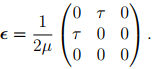(6.84)

In chapter 3, section 3.10.3, we showed that if the angle change between two line segments oriented along X and Y direction is κ, then this simple shearing deformation results in,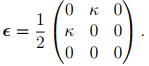(6.85)

Then, the shear modulus, G is defined as the ratio of the shear stress (τ ) to change in angle (κ) due to this applied shear stress between two orthogonal line elements in the plane of shear i.e.,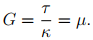(6.86)

Using equation (6.80b), the shear modulus can be written in terms of the Young’s modulus and Poisson’s ratio as,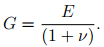(6.87)

Bulk Modulus
Consider a cuboid being subjected to uniform pure hydrostatic stress,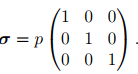(6.88)

Using (6.74) it could be seen that the above state of stress result in the strain tensor being,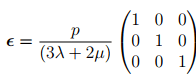(6.89)

The bulk modulus, K, is defined as the ratio of the mean hydrostatic stress to the volumetric strain when the body is subjected to pure hydrostatic stress. Thus,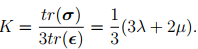(6.90)

Now, we would like to express the bulk modulus in terms of Young’s modulus and Poisson’s ratio. Towards this, we substitute equation (6.80) in (6.90) to obtain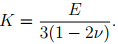(6.91)

The document Material Parameters Notes - Civil Engineering (CE) is a part of Civil Engineering (CE) category.
All you need of Civil Engineering (CE) at this link: Civil Engineering (CE)Use Code STAYHOME200 and get INR 200 additional OFF

Track your progress, build streaks, highlight & save important lessons and more!

,

,

,

,

,

,

,

,

,

,

,

,

,

,

,

,

,

,

,

,

,

;